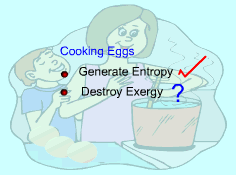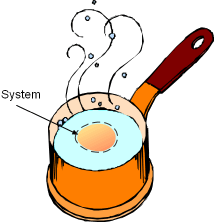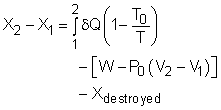Ch 7. Exergy Analysis Multimedia Engineering Thermodynamics Exergy ExergyChange ExergyBalance(1) ExergyBalance(2)
 Chapter 1. Basics 2. Pure Substances 3. First Law 4. Energy Analysis 5. Second Law 6. Entropy 7. Exergy Analysis 8. Gas Power Cyc 9. Brayton Cycle 10. Rankine Cycle Appendix Basic Math Units Thermo Tables Search eBooks Dynamics Fluids Math Mechanics Statics Thermodynamics Author(s): Meirong Huang Kurt Gramoll ©Kurt GramollTHERMODYNAMICS - CASE STUDY IntroductionProblem Description Pat is taking a thermodynamics course this semester and he tries to connect his daily life with what he learned in class. Recently, he has calculated how much entropy is generated when boiling eggs. Now, he wanders how much exergy is destroyed when the eggs are boiled. What is known: The 6-cm-diameter spherical egg at 10oC is dropped into boiling water. The boiling water is at 100oC. The egg has a density of 1,000 kg/m3 and a specific heat of 3.4 kJ/(kg-K). The ambient pressure is 1 bar and the ambient temperature is 25oC.Consider the Egg as a Closed System Question How much exergy is destroyed by boiling one egg to 100oC? Approach Take the egg as a closed system. Use the exergy balance of closed system to determine the exergy destroyed during cooking eggs. The exergy balance of closed system is